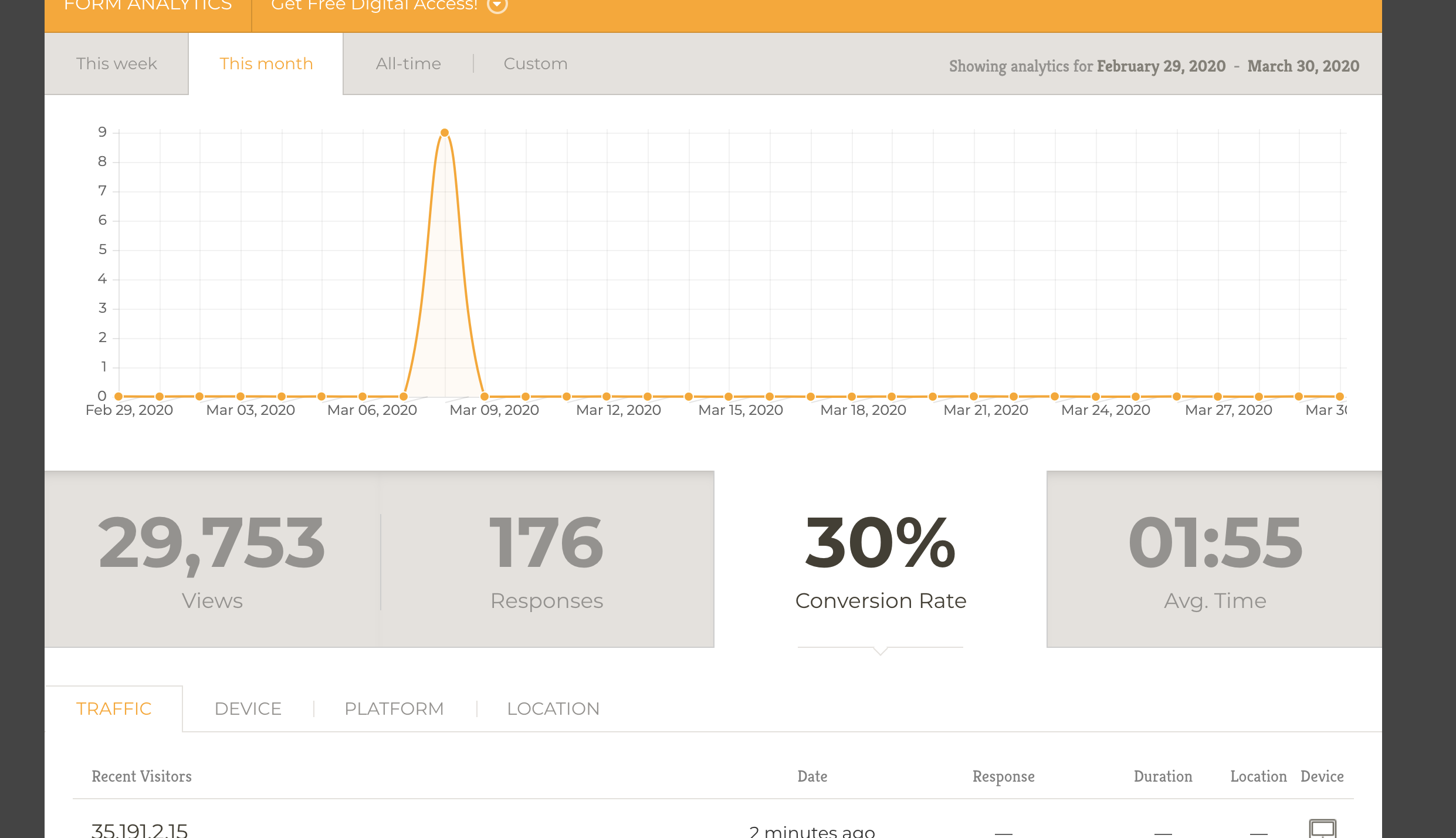# Form Analytics: How is the conversion rate calculated?

•ConcordiaSupply
Asked on March 30, 2020 at 01:29 PM

Hi there,

I'm trying to understand how analytics are calculated (e.g. conversion rates.).•